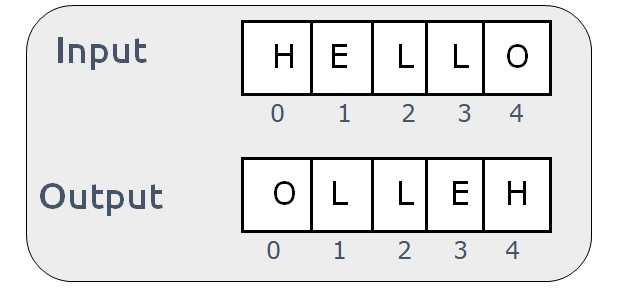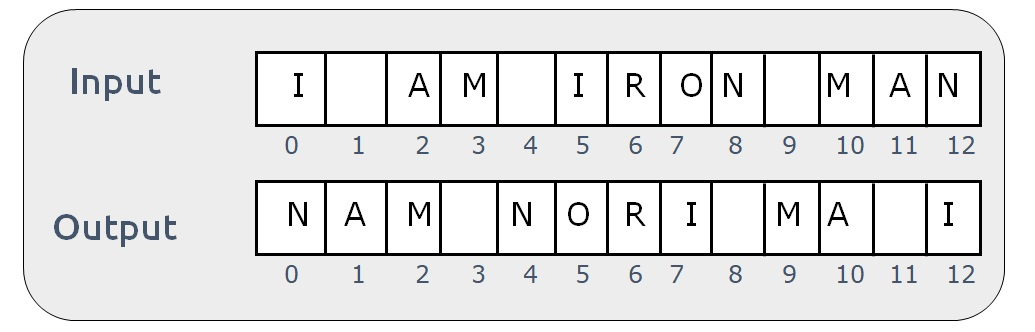# Reverse a String

Problem Statement: Reverse a String. Write a program that reverses a given string (in-place).

Examples:

``
``

Solution:

Disclaimer: Don’t jump directly to the solution, try it out yourself first.

Solution 1:

Solution 1: Using Stack

Pre-req: Stack Data Structure

Intuition: Stack is a Last-In-First-Out (LIFO) data structure. If we iterate over the string and push each character from start to end, the stack will contain the string in a reverse way.

Approach:

• Take an empty stack
• Iterate over the given string from start to end.
• In each iteration, push the character at index i to the stack.
• After the first loop is completed, set a while loop till the stack is non-empty.
• Pop the character at the stack and start re-assigning the string.

Dry Run:Code:

## C++ Code

``````#include <bits/stdc++.h>

using namespace std;

int main() {

string str = "HELLO";
stack < char > st;
int n = str.length();

for (int i = 0; i < n; i++) {
st.push(str[i]);
}

int j = 0;
while (!st.empty()) {
char elem = st.top();
st.pop();
str[j] = elem;
j++;
}

cout << "The reversed string is- " << str;

}
``````

Output: The reversed string is- OLLEH

Time Complexity: O(N)

Reason: We iterate for the length of the string two times.

Space Complexity: O(N)

Reason: We are using an external stack

## Java Code

``````import java.io.*;
import java.util.*;

class takeuforward {

// Driver code
public static void main(String[] args)
{
String str = "HELLO";

char[] reverseString = new char[str.length()];
Stack<Character> stack = new Stack<Character>();

for (int i = 0; i < str.length(); i++) {

stack.push(str.charAt(i));
}

int i = 0;
while (!stack.isEmpty()) {

reverseString[i++] = stack.pop();
}
// return string object
str=  new String(reverseString);

System.out.print("The reversed string is- "+ str);

}
}
``````

Output: The reversed string is- OLLEH

Time Complexity: O(N)

Reason: We iterate for the length of the string two times.

Space Complexity: O(N)

Reason: We are using an external stack

Solution 2: Using the two-pointer Approach

Intuition:

To reverse a string we need to think in terms of swapping the characters. The first index character needs to be swapped with the last character. Similarly, the second character needs to be swapped with the second last character and so on.

Approach:

The algorithm approach can be stated as:

• Set up two pointers i and j ( i=0 and j=(length of string -1)).
• Set up a while loop to iterate till i<j
• In every iteration swap the values at str[i] and str[j].
• Increment i and decrement j.

Dry Run:Code:

## C++ Code

``````#include <bits/stdc++.h>

using namespace std;

void swap(string & a, int i, int j) {
char temp = a[i];
a[i] = a[j];
a[j] = temp;
}

void reverseStr(string & a) {
int i = 0;
int j = a.length() - 1;

while (i < j) {
swap(a, i, j);
i++;
j--;
}
}

int main() {

string str = "HELLO";
reverseStr(str);
cout << "The reversed string is- " << str;

return 0;
}
``````

Output:

The reversed string is- OLLEH

Time Complexity: O(N)

Reason: The while loop will iterate n/2 time

Space Complexity: O(1)

Reason: We are not using any extra space.

## Java Code

``````import java.util.*;
import java.lang.*;
import java.io.*;

class Solution {
public static String swap(String s, int i, int j) {
StringBuilder str = new StringBuilder(s);
str.setCharAt(i, s.charAt(j));
str.setCharAt(j, s.charAt(i));

return str.toString();
}
public static void main(String[] args) {
String str = "HELLO";
int i = 0;
int j = str.length() - 1;

// reversing a String
while (i < j) {
str = swap(str, i, j);
i++;
j--;
}

System.out.print("The reversed string is- "+ str);
}
}
``````

Output:

The reversed string is- OLLEH

Time Complexity: O(N)

Reason: The while loop will iterate n/2 time

Space Complexity: O(1)

Reason: We are not using any extra space.

### Solution 3: Using library functions

There are in-built library functions that can be used to reverse a string. The following two functions can be used in C++ and Java respectively.Note: In Java, the String class does not have a reverse function but StringBuilder has, therefore we convert the given string to StringBuilder.

Code:

## C++ Code

``````#include <bits/stdc++.h>

using namespace std;

int main() {

string str = "HELLO";

reverse(str.begin(), str.end());

cout << "The reversed string is- " << str;

}``````

Output:

The reversed string is- OLLEH

Time Complexity: O(N)

Reason: The library function takes O(N) time

Space Complexity: O(1)

Reason: We are not using any extra space.

## Java Code

``````import java.util.*;

class takeuforward {
public static void main(String[] args)
{
String str = "HELLO";

StringBuilder str1 = new StringBuilder(str);
str1.reverse();

str=str1.toString();

// print reversed String
System.out.println("The reversed string is- "+str);
}
}
``````

Output:

The reversed string is- OLLEH

Time Complexity: O(N)

Reason: The library function takes O(N) time

Space Complexity: O(1)

Reason: We are not using any extra space.